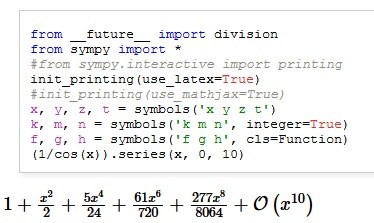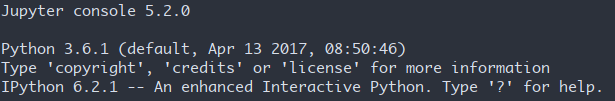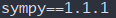# 如何使用emacs org python 直接输出mathjax直接可以渲染的代码?

emacs 就是这样，应该没有专职的 emacs 开发者，都是兴趣使然。用 emacs 的过程必然是折腾的过程。

(add-to-list 'load-path "d:/git/scimax/scimax")
'((ipython . t)
(python . t)
(emacs-lisp . t)
))
;config ipython
(require 'ob-ipython)
;;
(setq org-confirm-babel-evaluate nil)
;;
(defun org-babel-tangle-and-execute ()
(interactive)
(org-babel-tangle)
(org-babel-execute-buffer)
(org-display-inline-images))


(set-language-environment 'Chinese-GB)
(set-keyboard-coding-system 'euc-cn)
(set-clipboard-coding-system 'euc-cn)
(set-terminal-coding-system 'euc-cn)
(set-buffer-file-coding-system 'euc-cn)
(set-selection-coding-system 'euc-cn)
(modify-coding-system-alist 'process "*" 'euc-cn)
(setq default-process-coding-system '(euc-cn . euc-cn))
(setq-default pathname-coding-system 'euc-cn)


#+BEGIN_SRC ipython :results value :ob-ipython-results text/latex
from __future__ import division
from sympy import *
#from sympy.interactive import printing
init_printing(use_latex=True)
#init_printing(use_mathjax=True)
x, y, z, t = symbols('x y z t')
k, m, n = symbols('k m n', integer=True)
f, g, h = symbols('f g h', cls=Function)
(1/cos(x)).series(x, 0, 10)
#+end_src1 个赞